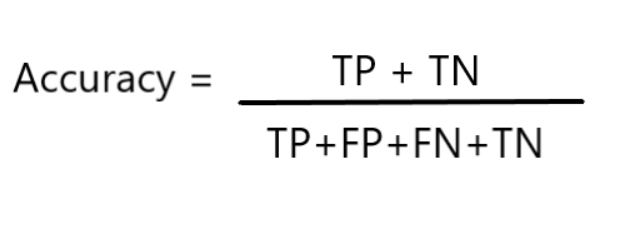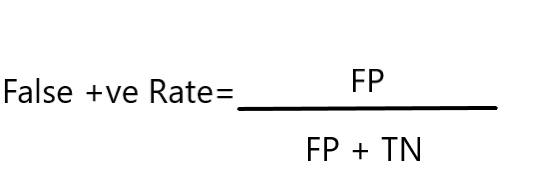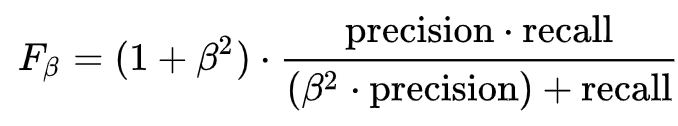# .css-4zleql{display:block;}Ashwin Sharma P# Confusion Matrix

Ashwin Sharma P
·Aug 15, 2020·

Today we are going to discuss some of the metrics in classification.

Let us first look at the confusion matrix.The Accuracy is calculated as:-The False Positive Rate is calculated as given:-Here;

TP:- True Positive TN:- True Negative FP:- False Positive FN:- False Negative

Consider a dataset with 1000 records of people who smoke and who do not smoke, which has the output as a binary classification. i.e., yes or no.

If the record has around 500 yes, 500 no or 600 yes, 400 no, we can consider it as a balanced dataset. But consider we have around 900 people who are smokers i.e., yes and only 100 people who are non-smokers i.e., no. It is a completely imbalanced dataset and predicted accuracy won't be matching with the actual value.

Due to this imbalanced dataset, the ML algorithms will become biased. In this case, we go for the usage of recall, precision and F-Beta to predict the accuracy.

Let us look what is precision, recall and F-Beta score now.

### Precision:-

Precision refers to the percentage of results which are relevant. In simpler words, it tells us, "out of the outputs which were predicted as positive, how many were actually positive."

A real case scenario where precision can be used is when a patient who does not have a disease is predicted as an infected person (i.e., False Positive value). Hence, in this case, our aim should be to minimize the value of FP. So, we use precision when False Positive value is important for our analysis.

### Recall:-

Recall refers to the percentage of total relevant results which were correctly classified by our algorithm. That is, out of the total positive values, how many were predicted as positive.

An example of this is when an infected person's test result is negative, which can lead to harmful consequences in a real-world scenario. Thus, here the False Negative value holds higher prominence and we should try to decrease this value. So we use recall when we have FN value as important.

### F-Beta Score:-

Given below is the formula to calculate the F-Beta score.Here the β value is selected based on whether the False Positive or False Negative value plays a major role in our data. If both are equally important, we consider β as 1.

If False Positive value has greater importance, β value is selected between 0 to 1 and if False Negative value has a greater importance β value ranges from 1 to 10.

The F-Beta score reaches its optimum value when β=1 and hence we generally hear people refer to it as F1-score instead of F-Beta score.